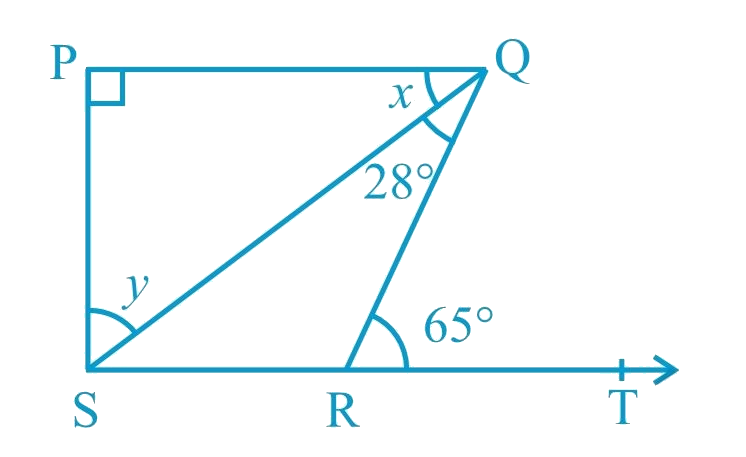In the verge of coronavirus pandemic, we are providing FREE access to our entire Online Curriculum to ensure Learning Doesn't STOP!

# Ex.6.3 Q5 Lines and Angles Solution - NCERT Maths Class 9

Go back to  'Ex.6.3'

## Question

In Fig. below, if $$PQ ⊥ PS$$ , $$PQ || SR$$ ,$$\angle SQR = 28^\circ$$ and $$\angle QRT = 65^\circ$$ ,then find the values of $$x$$ and $$y$$ .## Text Solution

What is known?

$$PQ ⊥ PS,\, PQ || SR$$,  $$\angle SQR = 28^\circ$$ and $$\angle QRT = 65^\circ$$

What is unknown?

$$x$$ and $$y$$.

Reasoning:

As we know when two parallel lines are cut by a transversal, alternate interior angles formed are equal.

Angle sum property of a triangle:

Sum of the interior angles of a triangle is $$360^\circ$$.

Steps:

Given,

$$PQ ⊥ PS,\, PQ || SR$$,  $$\angle SQR = 28^\circ$$ and $$\angle QRT = 65^\circ$$

\begin{align}\angle PQR &= \angle QRT\\ \begin{array}{I}(\text{Alternate }\end{array} &\begin{array}{I}\text{interior angles}) \end{array}\\\\ \angle PQS + \angle SQR &= \angle QRT \\ \text{(By figure)}\\\\x + 28^\circ &= 65^\circ \\x &= 65^\circ - 28^\circ \\x &= 37^\circ \end{align}

Now, in $$\Delta PQS$$

\begin{align}\angle PQS + \angle PSQ + \angle QPS &=180^\circ\\ ( \text{Angle sum property of a }& \text{triangle}\rm{.})\\ \\37^\circ + y + 90^\circ &= 180^\circ \\y &=180^\circ \!- \!127^\circ \\y &=\, 53^\circ \end{align}

Hence, $$x = 37^\circ$$ and $$y = 53^\circ$$

Learn from the best math teachers and top your exams

• Live one on one classroom and doubt clearing
• Practice worksheets in and after class for conceptual clarity
• Personalized curriculum to keep up with school